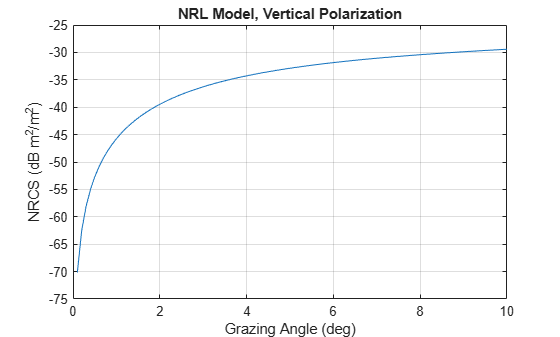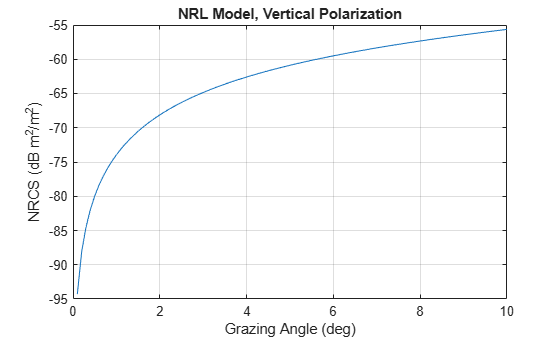# surfaceReflectivitySea

Normalized reflectivity of sea surface

## Description

Normalized reflectivity is the radar cross-section of a unit area of a sea surface. Multiplying by the total area of a surface or the illuminated area of a surface gives the total radar cross-section. Normalized reflectivity is also referred to as surface σ0 and is a function of frequency and grazing angle.

To compute the normalize reflectivity:

1. Create the `surfaceReflectivitySea` object and set its properties.

2. Call the object with arguments, as if it were a function.

## Creation

### Syntax

``refl = surfaceReflectivitySea``
``refl = surfaceReflectivitySea(Name=Value)``

### Description

example

````refl = surfaceReflectivitySea` creates a normalized reflectivity object, `refl`, for a sea surface. Use this object to generate a normalized radar cross section (NRCS). This syntax assumes a `'NRL'` sea model with a sea state of zero.```

example

````refl = surfaceReflectivitySea(Name=Value)` also creates a normalized reflectivity object for a sea surface with the specified property `Name` set to the specified `Value`. You can specify additional name-value pair arguments in any order as (`Name1=Value1`,...,`NameN=ValueN`).Example: ```refl = surfaceReflectivitySea(Model="Hybrid",SeaState=2,Speckle="Rayleigh")``` creates a normalized reflectivity object for a sea surface using the Hybrid model with a `SeaState` of 2 and a Rayleigh `Speckle` type.```

## Properties

expand all

Unless otherwise indicated, properties are nontunable, which means you cannot change their values after calling the object. Objects lock when you call them, and the `release` function unlocks them.

If a property is tunable, you can change its value at any time.

Sea reflectivity model, specified as `'NRL'`, `'APL'`, `'GIT'`, `'Hybrid'`, `'Masuko'`, `'Nathanson'`, `'RRE'`, `'Sittrop'`, `'TSC'`, or `'ConstantGamma'`. The table.Sea Reflectivity Models summarize the sea surface models available in the radar simulation and their domain of application

Sea state, specified as a nonnegative integer from 0 - 8.

Data Types: `double`

Polarization of reflectivity model, specified as `'H'` for horizontal polarization or `'V'` for vertical polarization.

#### Dependencies

To enable this property, set the `Model` property to any value except `'ConstantGamma'`.

Sea gamma value used in the constant gamma clutter reflectivity model, specified as a scalar. The gamma value depends on both sea state and the operating frequency. Units are in dB.

Example: -25

#### Dependencies

To enable this property, set the `Model` property to `ConstantGamma`.

Data Types: `double`

Speckle distribution type, specified as `'None'`, `'Lognormal'`, `'Rayleigh'`, `'Weibull'`, or `'Custom'`. Speckle is a multiplicative factor used to make clutter data appear noisier and is especially applicable to imaging applications.

Speckle is correlated with clutter RCS and is applied as I = σ*n, where σ represents the clutter RCS and n represents random numbers, which are often drawn from an independent identically-distributed unity mean noise statistical distribution.

• `None` – No speckle is applied.

• `Lognormal` – Speckle has a lognormal distribution. Define the distribution using the `SpeckleMean` and `SpeckleStandardDeviation` properties. Default values of these properties create speckle with a normalized mean lognormal distribution.

• `Rayleigh` – Speckle has a Rayleigh distribution. Define the distribution using the `SpeckleScale` property. The default value of this property creates speckle with a unit mean Rayleigh distribution.

• `Weibull` – Speckle has a Weibull distribution. Define the distribution using the `SpeckleScale` and `SpeckleShape` properties. The default values of these properties create speckle with a unit mean Rayleigh distribution.

Data Types: `char` | `string`

Mean value of lognormal-distributed speckle, specified as a scalar.

#### Dependencies

To enable this property, set the `Speckle` property to `'Lognormal'`.

Data Types: `double`

Standard deviation of lognormal-distributed speckle, specified as a non-negative scalar.

#### Dependencies

To enable this property, set the `Speckle` property to `'Lognormal'`.

Data Types: `double`

Scale parameter for speckle for the Rayleigh and Weibull distributions, specified as a positive scalar.

#### Dependencies

To enable this property, set the `Speckle` property to `'Rayleigh'` or `'Weibull'`.

Data Types: `double`

Shape value for the Weibull speckle distribution, specified as a positive scalar.

#### Dependencies

To enable this property, set the `Speckle` property to `Weibull`.

Data Types: `double`

## Usage

### Syntax

``nrcs = refl(graz,freq)``
``nrcs = refl(graz,freq,lookang)``

### Description

example

````nrcs = refl(graz,freq)` returns the normalized radar cross section `nrcs` at grazing angle `graz` and frequency `freq`.```
````nrcs = refl(graz,freq,lookang)` also specifies the radar look angle `lookang` with respect to the wind direction. To enable this syntax, set the `Model` property to `'APL'`, `'GIT'`, `'Hybrid'`, `'Masuko'`, `'Sittrop'`, or `'TSC'`.```

### Input Arguments

expand all

Grazing angle of surface relative to radar, specified as a scalar or a length-M row vector of nonnegative values. Grazing angles must lie between 0° and 90°. Units are in degrees.

Transmitted frequencies, specified as a positive scalar or N-length vector of positive values. Units are in Hz.

Example: `freq = 7*10e9`

Look angle with respect to wind direction, specified as a scalar between 0° and 180°. The look angle is zero when looking upwind.

#### Dependencies

To enable this argument, set the `Model` property to `'APL'`, `'GIT'`, `'Hybrid'`, `'Masuko'`, `'Sittrop'`, or `'TSC'`.

Data Types: `double`

### Output Arguments

expand all

Normalized surface reflectivity, returned as either a real-valued N-length row vector or a real-valued M-by-N matrix. Normalized reflectivity is also called normalized radar cross section. M is the length of the grazing angle or depression angle vector `graz` and N is the length of the frequency vector `freq`. `nrcs` is dimensionless but often expressed as m²/m².

## Object Functions

To use an object function, specify the System object™ as the first input argument. For example, to release system resources of a System object named `obj`, use this syntax:

`release(obj)`

expand all

 `step` Run System object algorithm `release` Release resources and allow changes to System object property values and input characteristics `reset` Reset internal states of System object

## Examples

collapse all

Create a sea surface normalized reflectivity object using the default NRL model and a sea state of 6. Obtain the normalized reflectivity at a frequency of 1 GHz over grazing angles from 0.1 to 10 degrees and assume vertical polarization. Plot the normalize reflectivity as a function of grazing angle.

```grazAng = 0.1:0.1:10; freq = 1e9; seastate = 6; pol = 'V'; refl = surfaceReflectivitySea(SeaState = seastate,Polarization = pol); nrcs = refl(grazAng,freq); plot(grazAng,pow2db(nrcs)) grid on xlabel('Grazing Angle (deg)') ylabel('NRCS (dB m^2/m^2)') title('NRL Model, Vertical Polarization')```Create a sea surface normalized reflectivity object using the default model parameters. Obtain the normalized reflectivity at a frequency of 1 GHz over grazing angles from 0.1 to 10 degrees and assume vertical polarization. Plot the normalize reflectivity as a function of grazing angle.

```grazAng = 0.1:0.1:10; freq = 1e9; refl = surfaceReflectivitySea```
```refl = surfaceReflectivitySea with properties: Model: 'NRL' SeaState: 1 Polarization: 'H' Speckle: 'None' ```
```nrcs = refl(grazAng,freq); plot(grazAng,pow2db(nrcs)) grid on xlabel('Grazing Angle (deg)') ylabel('NRCS (dB m^2/m^2)') title('NRL Model, Vertical Polarization')```Configure a `radarscenario` to simulate a reflective sea surface. Add a sea surface object to define the physical properties of the scenario surface. The surface is a simple 400-by-400 meter rectangle. Use the `surfaceReflectivitySea` function to create a GIT model with a sea state 3. Then, use the scenario `seaSurface` method to add the rectangular sea region and the radar reflectivity model to the scenario. Use a surface reference height of 16 meters.

```scene = radarScenario(UpdateRate = 0, IsEarthCentered = false); refl = surfaceReflectivitySea(Model = "GIT", SeaState = 3, Polarization = "V"); srf = seaSurface(scene,RadarReflectivity = refl, ... Boundary=[-200 200; -200 200],ReferenceHeight = 16)```
```srf = SeaSurface with properties: WindSpeed: 10 WindDirection: 0 Fetch: Inf SpectralModel: [] RadarReflectivity: [1×1 surfaceReflectivitySea] ReflectivityMap: 1 ReferenceHeight: 16 Boundary: [2×2 double] ```

expand all

 Gregers-Hansen, V. and Mittal, R. "An Improved Empirical Model for Radar Sea Clutter Reflectivity." NRL/MR/5310-12-9346, Apr. 27, 2012.

 Barton, David Knox. Radar Equations for Modern Radar. Artech House, 2013.

 Reilly, J. P., R. L. McDonald, and G. D. Dockery. "RF-Environment Models for the ADSAM Program." Report No. A1A97U-070, Laurel, MD: Johns Hopkins University Applied Physics Laboratory, August 22, 1997.

 Ward, Keith D., Simon Watts, and Robert J. A. Tough. Sea Clutter: Scattering, the K-Distribution and Radar Performance. IET Radar, Sonar, Navigation and Avionics Series 20. London: Institution of Engineering and Technology, 2006.

 Antipov, Irina. "Simulation of Sea Clutter Returns." Department of Defence, June 1998.

 Masuko, Harunobu, Ken'ichi Okamoto, Masanobu Shimada, and Shuntaro Niwa. "Measurement of Microwave Backscattering Signatures of the Ocean Surface Using X Band and K a Band Airborne Scatterometers." Journal of Geophysical Research 91, no. C11 (1986): 13065. https://doi.org/10.1029/JC091iC11p13065.

 Nathanson, Fred E., et al. Radar Design Principles: Signal Processing and the Environment. 2. ed., Repr, Scitech Publ, 2004.